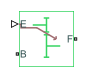# Simple Gear with Variable Efficiency

Gear set with parallel-axis rotation and variable meshing efficiency

•Libraries:
Simscape / Driveline / Gears

## Description

The Simple Gear with Variable Efficiency block represents a simple gear train with variable meshing efficiency. The gear train transmits torque at a specified ratio between base and follower shafts arranged in a parallel configuration. Shaft rotation can occur in equal or opposite directions. Gear losses are optional. They include meshing and viscous bearing losses. To specify the variable meshing efficiency, the block contains a physical signal port that you can use to input a general time-varying signal. Inertia and compliance effects are ignored.

### Simple Gear Model

Ideal Gear Constraint and Gear Ratio

Simple Gear imposes one kinematic constraint on the two connected axes:

 rFωF = rBωB . (1)

The follower-base gear ratio gFB = rF/rB = NF/NB. N is the number of teeth on each gear. The two degrees of freedom reduce to one independent degree of freedom.

The torque transfer is:

 gFBτB + τF – τloss = 0 , (2)

with τloss = 0 in the ideal case.

### Nonideal Gear Constraint and Losses

In the nonideal case, τloss ≠ 0. For general considerations on nonideal gear modeling, see Model Gears with Losses.

### Thermal Model

You can model the effects of heat flow and temperature change by enabling the optional thermal port. To enable the port, set Friction model to ```Temperature-dependent efficiency```.

### Variables

Use the Variables settings to set the priority and initial target values for the block variables before simulating. For more information, see Set Priority and Initial Target for Block Variables.

## Limitations

• Gear inertia is assumed to be negligible.

• Gears are treated as rigid components.

## Ports

### Input

expand all

Physical signal input port associated with the meshing efficiency of the gear. The block accepts efficiency in the range (0,1].

### Conserving

expand all

Mechanical rotational conserving port associated with the base shaft.

Mechanical rotational conserving port associated with the follower shaft.

Thermal conserving port associated with heat flow. Heat flow affects gear temperature, and therefore, power transmission efficiency.

#### Dependencies

To enable this port, set Thermal port to `Model`.

## Parameters

expand all

### Main

Gear ratio. This is the fraction of follower over base gear teeth numbers, NF/NB. The ratio must be positive.

Relative rotation between shafts. This is the rotation direction of the output shaft with respect to the input shaft. You can choose either equal or opposite rotational directions.

### Meshing Losses

Smallest efficiency value allowed for the gear. The efficiency is the power ratio between output and input shafts. The physical signal input is maintained at values below the minimum efficiency or above a value of 1. The value must be positive.

Follower shaft power above which full efficiency factor is in effect. A hyperbolic tangent function smooths the efficiency factor between zero, when at rest, and the efficiency set point.

### Viscous Losses

Two-element array with the viscous friction coefficients in effect at the base and follower shafts. The default array corresponds to zero viscous losses.

### Thermal Port

Whether to model thermal effects and enable port H for temperature-dependent performance.

Thermal energy required to change the component temperature by a single degree. The greater the thermal mass, the more resistant the component is to temperature change.

#### Dependencies

To enable this parameter, set Thermal port to `Model`.

Component temperature at the start of simulation. The initial temperature alters the component efficiency according to an efficiency vector that you specify, affecting the starting meshing or friction losses.

#### Dependencies

To enable this parameter, set Thermal port to `Model`.

## Version History

Introduced in R2013a